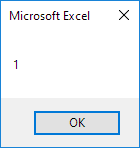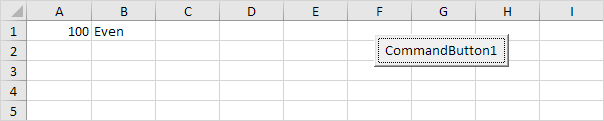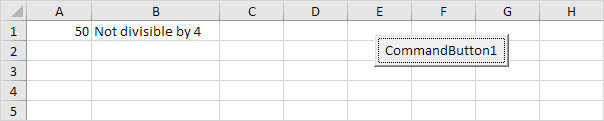# Mod Operator

The Mod operator in Excel VBA gives the remainder of a division. This page starts with some simple examples.

### Mod 101

Place a command button on your worksheet and add the following code line:

MsgBox 7 Mod 2

Result when you click the command button on the sheet:Explanation: 7 divided by 2 equals 3 with a remainder of 1.

Code line:

MsgBox 8 Mod 2

Result:Explanation: 8 divided by 2 equals 4 with a remainder of 0.

### Even or Odd

Let's create a program that uses the Mod operator to check if a number is even or odd.

Code lines:

Dim x As Integer
x = Range("A1").Value

If x Mod 2 = 0 Then
Range("B1").Value = "Even"
Else
Range("B1").Value = "Odd"
End If

For example, enter the value 100 into cell A1 and click the command button on the sheet.

Result:Explanation: a number is even if it is divisible by 2 without a remainder.

### Divisible by Another Number

Let's create a program that uses the Mod operator to check if a number is divisible by another number.

Code lines:

Dim x As Integer
x = Range("A1").Value

If x Mod 4 = 0 Then
Range("B1").Value = "Divisible by 4"
Else
Range("B1").Value = "Not divisible by 4"
End If

For example, enter the value 50 into cell A1 and click the command button on the sheet.

Result:Explanation: 50 divided by 4 equals 12 with a remainder of 2. In other words, 50 is not divisible by 4.

Go to Next Chapter: Loop